# PHP Functions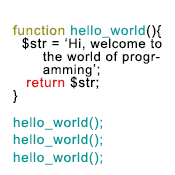## Why function is necessary?

In your project, sometimes you need to perform same task multiple times. Therefore, you need to write the same code every time it requires. It makes programming time consuming, boring, and tedious. To make development easier, a programming feature named function comes to rescue in this stage. You can define the set of statements that perform a single task once as a function and call it by its name whenever you need.

One single task is defined in one standard function.

## What is a function?

A function is an independent named block of codes that

• is defined to perform a single task.
• can be called from another part of the program, and
• may or may not return a value.

Codes inside a function are not executed until the function is called.

• Fewer codes: A function is written only once and can be called as many times as it requires.
• Less execution time: A function can be called many times in a page but it is compiled only once.
• Fewer bugs: As a function is written only once and removes the need to write the codes for the same task every time it requires, it reduces number of bugs.

## Defining a Function

Syntax

function function_name(parameter_1, parameter_2, …, parameter_n){
// statements
}

• Function definition starts with the keyword function.
• Then function name comes.
• Function name is followed by a pair of parentheses which contain optional parameters (parameters are input to the function and are used inside the function. More about parameters in a bit). Parameters are separated by commas if there is more than one parameter.
• Finally, a pair of curly braces that contains statements. The statements perform the task for which the function is defined. The optional parameter(s) are used here.

Example 1:

```<?php
function welcome(){
echo “Hi, welcome to Schools of Web.”;
}
?>```

When the above function is called it will perform one task – it prints the sentence – “Hi, welcome to Schools of Web.”. The function has no parameter.

Example 2:

```<?php
function concat_names(\$f_name, \$l_name){
echo \$f_name . “ ” . \$l_name;
}
?>```

When the above function is called it will take 2 parameters – Sfirst_name and \$last_name and perform one task  – concatenating Sfirst_name and the \$last_name, it prints that.

## Function naming rules:

• Function name should be meaningful so that it indicates what the function does.
• A valid function name must starts with a letter or underscore followed by any number of letters, numbers, or underscores.
• Every function name should be unique.
• Function names are case insensitive. That means Welcome, WELCOME, WelcomE are same function name.

## Calling a function

Calling a function is simple – just write the name of the function followed by a pair of parentheses.

Syntax:
function_name();

• After calling a function, the statements inside it are executed.
• After the function execution is completed, the program control returns to the point where the function was called.Example 1:

In the line no 4 in the example below, the function example() is called.

```<?php
function welcome(){
echo “Hi, welcome to Schools of Web.”;
}
welcome(); // Function welcome() is called here.
?>```

Output:
Hi, welcome to Schools of Web.

Explanation:

The function welcome() is defined in line 1-3. In this stage nothing happens. When the function is called at line 4, the interpreter finds it at line no. 1 and enters into it, executes the statement(s) inside it. Here it prints the sentence “Hi, welcome to Schools of Web.” in line no 2.

Example 2:

In the line no 4 in the example below, the function concat_names() is called which has two parameters – \$f_name and \$l_name.

```<?php
function concat_names(\$f_name, \$l_name){
echo \$f_name . “ ” . \$l_name;
}
concat_names(“Neil”, “Fin”);
?>```

Output:
Neil Fin

Explanation:
The function concat_names() is defined in line 1-3. In this stage nothing happened. The function is called in line 4 and passes two values-“Neil” and“Fin” to the function. The interpreter finds the function at line 1 and sets “Neil” in the variable \$f_name and “Fin” in the variable \$l_name. The function can use those variables inside the curly braces. It prints the Neil Fin in line no. 2.

## What are arguments and parameters?

When you call a function that accepts inputs, you enter values separated by commas like this-

```<?php
concat_names(“Neil”, “Fin”);
?>```

These values are called arguments.On the other hand, the arguments are sent to a list of inputs separated by commas within the parentheses in the function definition. These inputs are called parameters. The receiving function can use those parameters inside the curly braces.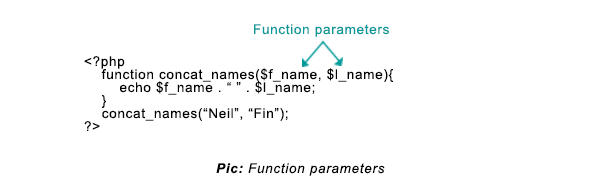When a function which has arguments is called, it passes the arguments to the corresponding parameters of the receiver function –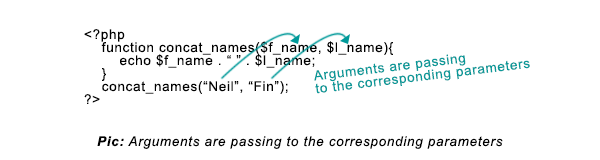When a caller function has more number of arguments than the number of parameters of the receiving function, the extra arguments are ignored-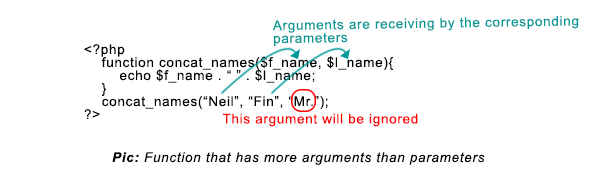When a caller function has fewer number of arguments than the number of parameters of the receiving function, it will generate a warning of missing arguments-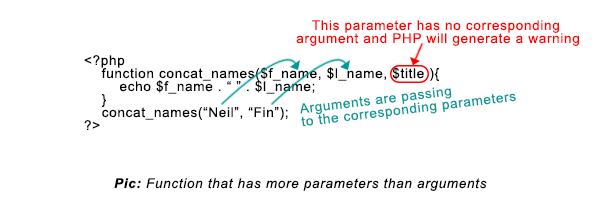## Passing arguments

You can pass arguments to a function in two ways-

1. By value
2. By reference

## Passing arguments by value

Look at the following example-

```<?php
function square(\$number){
\$number = \$number * \$number;
}
\$number = 3;
square(\$number);
echo \$number;
?>```

Output:
3

In the above example, you’re passing arguments by values in line no. 5. The variable \$number occupies the value. Here are what happen when you pass arguments by value

1. A new variable is created in the function containing the value that is passed by the argument even the names of the variable are identical. In the example, the new variable \$number is created at line 1 with the value of 3 when the function square() is called in line no. 5. Remember, Variable \$number at line 5 are different than the variable at line 1.
2. The new variable created in the function dies when the function ends. So, any changes of this new variable inside the function will not affect on the original variable. That’s why the above script output the value of \$number at line 6 as 3 that is defined at line 4.

## Passing arguments by reference

If you want to change the value of the original variable by changing its value inside the function, then you need to pass the argument by reference. Check the following example-

```<?php
function square(&\$number){
\$number = \$number * \$number;
}
\$number = 3;
square(\$number);
echo \$number;
?>```

Output:
9

How passing arguments by reference works:

• To pass an argument as reference places an ampersand before the parameter. In the above example the argument \$number at line 5 is passed by reference to the function at line 1, so the ampersand (&) is placed before the \$number. Here, it’s just a reference of the original variable.
• Any changes makes in the value of the variable inside the function will reflect in the original variable.
• As the value of the variable \$number is 3, so 3 is multiply by 3 and 9 is stored in the same variable \$number in line 3. As the change also reflects in the original variable, so the line 7 prints 9.

## Default parameters

When you define a function you can assign value of one or more parameters. If you don’t assign the argument(s) when you call a function that has parameters, the default value will work. On the other hand, if you assign the argument(s), the parameters will be replaced by the arguments. Few rules about the default parameters-

1. Default parameter(s) must be placed at last before the parameters have no default value.
2. As value of the default parameters, it must be either string or number.

Example:

```<?php
function square(\$number=5){
echo \$number = \$number * \$number;
}
square();
?>```

Output:
25

Explanation:
Though the function square() expects a parameter but no arguments is passed when calling at line 5. So the default value 5 is assigned \$number, hence the output 25.

## The return statement

A function not only can accept values but it also can return one or more values.

The return statement does two things-

1. Returns one or more values to the caller function and sends back the program control to the caller function.
2. Exits the function after the return statement and ignores the subsequent statements after the return statement if there is any.

Syntax

function function_name(){
// statements;
return \$value;
//statement_n;
}

• The above function returns “\$value” to the caller.
• The statements (here, statement_n) after the return will not be executed.

## Returning a value

The following function returns a single value-

```<?php
function welcome(){
return “Hi, welcome to Schools of Web.”;
echo ”Monday”;
}
welcome();
?>```

Output:

Explanation:
The welcome() function is called in line no. 5. After calling the welcome() function, it returns the string “Hi, welcome to Schools of Web.” from line no. 2 to the caller at line no. 5. Note, It will not output anything as no echo is used before the caller. The string simply gets back to the caller.

Please also note that the function will not print the string “Monday” in the screen as after return statement at line no. 2, the control back to the caller.

You can do two things with the returned value-

• You can simply print that
• You can assign it to a variable for further use.

Example:

```<?php
function welcome(){
return “Hi, welcome to Schools of Web.”;
echo ”Monday”;
}
echo welcome();
echo “<br />”;
\$val = welcome();
?>```

Output:
Hi, welcome to Schools of Web.

Explanation:
The welcome() function is called two times.

• In line no. 5, the return value is printed to the screen.
• In line no. 7, the return value is assigned to the \$val variable using the assignment variable that we can use later.

## Returning multiple values

To return multiply values from a function, you can use array. Check the following example-

The following function returns a single value-

```<?php
function day_name(){
\$day1=”Monday”;
\$day2=”Tuesday”;
\$day3=”Wednesday”;
return array(\$day1, \$day2, \$day3);
}
\$days = day_name();
echo \$days.” ”.\$days.” ”.\$days;
?>```

Output:

Monday Tuesday Wednesday

Explanation:

1. Function day_name() creates an array that has three elements in it in line no. 5 and returns to its caller.
2. When the function is called in line no. 7, the function returns the array to the caller assigns it in the \$days variable.
3. The next line prints the values of the array elements.

## Alternate ways of receiving multiple values

To assign the array elements in individual variables of your choice, you can use a built-in function – list(). PHP has lots of built-in variables to make web development easy. For now, just know that list() is used to assign some values to a list of variables at a time. The previous example can be rewritten with list() function as follows-

```<?php
function day_name(){
\$day1=”Monday”;
\$day2=”Tuesday”;
\$day3=”Wednesday”;
return array(\$day1, \$day2, \$day3);
}
list(\$day_1, \$day_2, \$day_3) = day_name();
echo \$day_1.” ”.\$day_2.” ”.\$day_3;
?>```

Output:

Monday Tuesday Wednesday

 ‹‹ PHP File-Inclusion : Previous Lesson Next Lesson: PHP Variable Scope ››